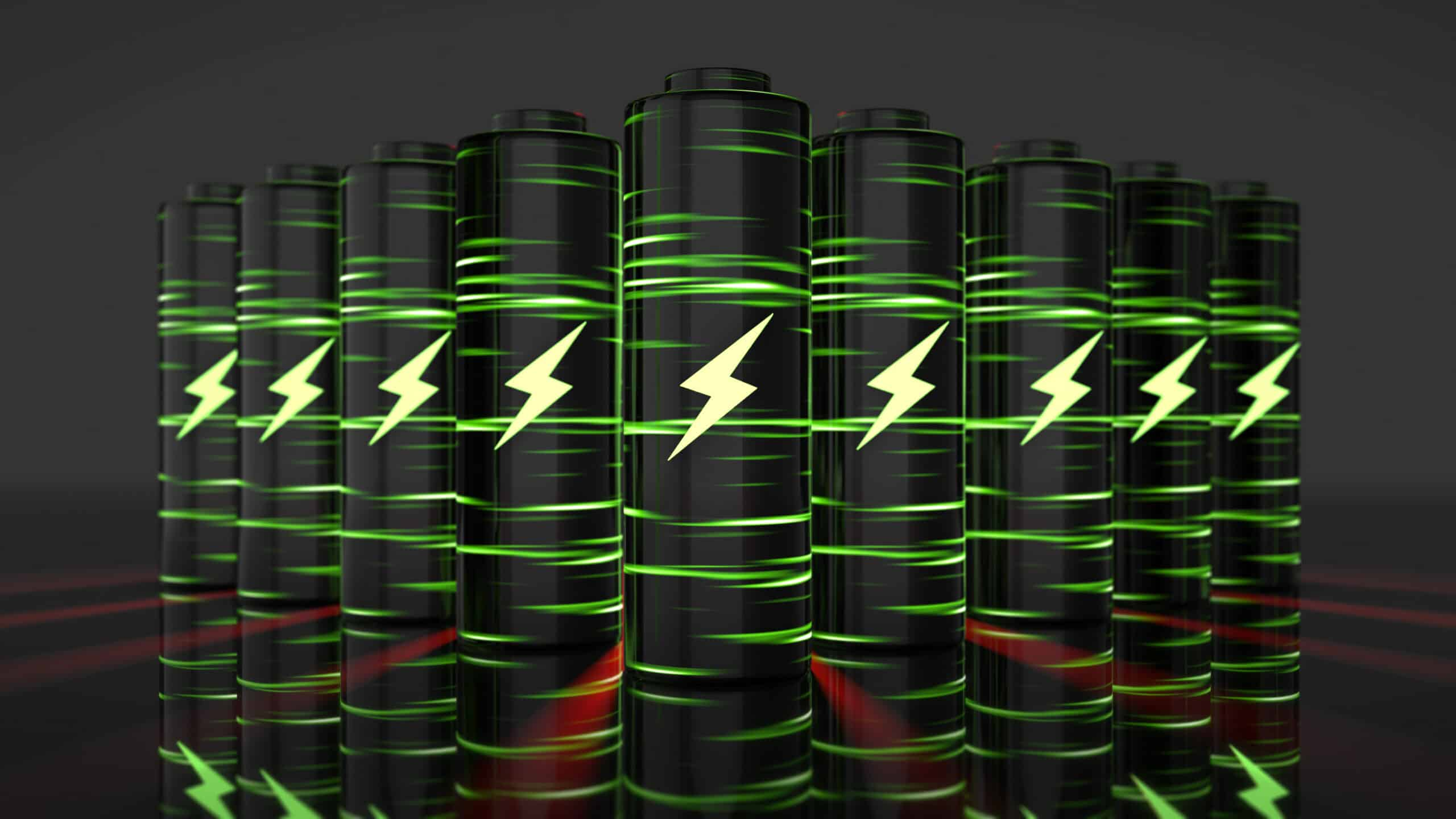Home

›

Articles

›

Concepts

›

Vs.

›

Kilowatt Hour vs. Amp Hour: What’s the Difference?# Kilowatt Hour vs. Amp Hour: What’s the Difference?

Most of the time, people are conscious of electricity and its effects. Especially considering how it lights up our homes, defrosts our food, and displays colorful images. But as far as how electricity operates, and the measurements used, it can get a little more complicated. It is important to understand the difference, especially when taking on a project involving electricity. So what is the exact difference between Kilowatt hour and Amp hour?

## Kilowatt Hour vs. Amp Hour: In-Depth Comparison

Now that we have explained the difference between kilowatt hours and amp hours, we can take a deep dive into what separates them.

### Definition of Kilowatt Hour:

To put it simply, a kilowatt hour is a unit of energy. For every kilowatt, there is one hour of power. One kilowatt hour equals 3.6 megajoules. Often, electrical companies will use kilowatts hours as a billing unit for electrical energy delivered to clients.

A more in-depth explanation is that a kilowatt hour is the total amount of energy used equaling to one kilowatt that is sustained for one or more hours. In most homes, people have more than one electrical product plugged into the wall, withdrawing energy. So instead of electrical companies trying to count up each time a product was plugged into the wall, and for how long, and then tracking the expense, electrical companies will supply the house with electricity and then (usually) at the end of the month the company will send the consumer a bill of how many kilowatt hours in total they used during that month.

### Definition of Amp Hour:

An amp hour or ampere-hour is a unit of electrical charge. Specifically, it is the amount of power that a battery can provide over an hour. So instead of how much energy was used over an hour, which is defined with Kilowatt, an amp hour is how much constant stored flow an object can provide over an hour.

Commonly, we see amp hours used to market products like car batteries, phones, and AA batteries. Usually, when there is a higher amp number on a product, it means that we can use the product for longer. However, that time can also depend on what the battery is supplying energy to. If the draw on the product is higher, then the battery will not last as long as it would for a lower-draw product.

## Kilowatt Hour vs. Amp Hour: 5 Must-Know Facts

• Amps use the symbol A, while watts use the symbol W.
• Amps measure the rate of an electric current, whereas watts measure the rate of energy transfer.
• When measuring amps use an ammeter, whereas for watts use a wattmeter.
• The average smartphone battery usually contains between 2,500 and 4,000 milliampere hours of electric capacity.
• Car batteries normally contain about a 50 ampere-hour battery capacity.

## Kilowatt Hour vs. Amp Hour: Which One Is Better? Which One Should You Use?

Whether you should use kilowatt hour or amp hour will depend on what your electric project entails. If you are working on a project that depends on knowing what the exact electric transfer is, then you would want to use Kilowatt hours. But if, for instance, your project depends on knowing how much flow is used from a stored capacity, then amp hour would be the best for you. With that being said, understanding what the terms kilowatt and amp hour means will greatly assist you in achieving all your electric project needs.

### Conclusion

In conclusion, although it may be confusing at first to notice the difference between kilowatt and amp hour, once we break it down into its core components, we understand the necessity for the different vocabulary. Now, because you understand the difference, you will be able to accomplish your electrical goals. But remember, always make sure when working on an electrical project that you take all the necessary precautions to prevent harm to both yourself and others.

What is the difference between a Kilowatt and a Kilogram?

The difference between a kilowatt and a kilogram is that a kilowatt refers to electricity, while a kilogram refers to weight. The prefix kilo in both these words means thousand to the total measurements, whereas the base word is what the Thousand is referring to. In this instance, a kilogram refers to 1,000 grams.

Are kilowatt and kilowatt hour the same?

Yes, and no. The word kilowatt refers to a unit of measurement in terms of electricity. Whereas kilowatt hour refers to the measurement of electricity over the course of an hour.

How much energy is stored in one kilowatt hour?

One kilowatt hour stores 3,600,000 joules of energy. Because the number of joules is so big, electric companies use kilowatt hours so as to not scare away their consumers.

Is an amp and an amp hour the same?

One refers to the other. What this means is that an amp hour refers to the unit of energy that would be measured over the course of an hour.

How much power is stored in one amp hour?

One amp hour using 12 volts will store 12 watts of energy. For reference, the average smartphone battery usually contains between 2,500 and 4,000 milliampere hours of electric capacity. Car batteries normally contain about a 50 ampere-hour battery capacity.